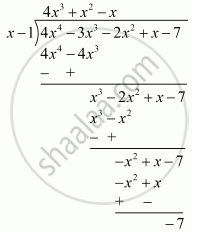Advertisement Remove all ads

# F(X) = 4x4 − 3x3 − 2x2 + X − 7, G(X) = X − 1 - Mathematics

Answer in Brief

f(x) = 4x4 − 3x3 − 2x2 + x − 7, g(x) = x − 1

Advertisement Remove all ads

#### Solution

Let us denote the given polynomials as

f (x) = 4x^4 - 3x^3 - 2x^2 + x - 7

g(x) = x-1

We have to find the remainder when f(x) is divided byg(x).

By the remainder theorem, when f(x) is divided by g(x) the remainder is

f(1) = 4(1)^4 - 3(1)^3 - 2(1)^2 + 1-7

 = 4 - 3- 2 + 1- 7

 = -7

Now we will show remainder by actual divisionSo the remainder by actual division is −7

Is there an error in this question or solution?
Advertisement Remove all ads

#### APPEARS IN

RD Sharma Mathematics for Class 9
Chapter 6 Factorisation of Polynomials
Exercise 6.3 | Q 2 | Page 14
Advertisement Remove all ads

#### Video TutorialsVIEW ALL 

Advertisement Remove all ads
Share
Notifications

View all notifications

Forgot password?
Course